ICSE Class 10 Study of Compounds B. Ammonia￼ Solution New

## ICSE Class 10 Study of Compounds B. Ammonia Solution

ICSE Class 10 Metallurgy Solution New Pattern

## Best Reference Books For Class 10 ICSE

Best Reference Books For Class 10 ICSE Physics Is OSWAL GURUKUL

## Chapter 9 - Study of Compounds B. Ammonia Exercise Intext 1

Question 1(a)

State the type of bonding present in ammonia, show by a diagram?Solution 1(a)

Covalent bonding is present in ammonia.

Question 1(b)

What is the formula of liquid ammonia? Account for the basic nature of this compound.Solution 1(b)

Formula of liquid ammonia is: NH3.

Liquid ammonia is liquefied ammonia and is basic in nature. It dissolves in water to give ammonium hydroxide which ionizes to give hydroxyl ions.

NH3 + H2NH4OH

NH4OH NH4++ OH-

Therefore it turns red litmus blue and phenolphthalein solution pink.Question 2

(a) Write a balanced chemical equation for the lab preparation of ammonia.

(b) How is ammonia dried and collected in the laboratory?

(d) Ammonia cannot be collected over water. Give reason.Solution 2

(a) Lab preparation of ammonia:

2NH4Cl+Ca(OH)2 CaCl2 +2H2O +2NH3

(b) The ammonia gas is dried by passing through a drying tower containing lumps of quicklime (CaO).

(d) Ammonia is highly soluble in water and therefore it cannot be collected over water.Question 3

(a) Explain with a diagram the preparation of aqueous ammonia.

(b) Name a drying agent for ammonia. Why are other drying agents such as P2O5 and CaCl2 not used to dry NH3Solution 3

(a) An aqueous solution of ammonia is prepared by dissolving ammonia in water. The rate of dissolution of ammonia to water is very high.

Diagram:

(b)The drying agent used is CaO in case of ammonia.

Other drying agents like P2O5 and CaCl2 are not used. As ammonia being basic reacts with them.

6NH3 + P2O5 +3H22(NH4)3PO4

CaCl2 +4NH3 CaCl2.4NH3Question 4

A substance 'A' was heated with slaked lime and a gas 'B' with pungent smell was obtained. Name the substances A and B and give a balanced equation.Solution 4

The substance A is Ammonium chloride and 'B' is Ammonia.

Reaction:

2NH4Cl + Ca(OH)2 CaCl2 + 2H2O + 2NH3Question 5

Ammonia is manufactured by Haber Process.

(a) Under what conditions do the reactants combine to form ammonia? Give a balanced equation for the reaction.

(b) In what ratio by volume, are the above gases used?

(c) State one possible source of each reactant used in Haber process.

(d) State whether the formation of ammonia is promoted by the use of high pressure or low pressure?

(e) Mention two possible ways by which ammonia produced is removed from unchanged gases.

(f) What is the function of:

(i) Finely divided iron

(ii) Molybdenum in the above process

(g) What is the percentage formation of ammonia?

(h) How can this percentage formation be increased?
Solution 5

(a) Conditions for reactants to combine :

Optimum temperature is 450o-500oC

Above 200 atm pressure

Finely divided iron as catalyst

Traces of molybdenum or Al2O3 as promoters.

Reaction:N2 +3H2 2NH3 + heat

(b) Dry nitrogen and dry hydrogen in the ratio of 1:3 by volume is made to combine.

(c) Source of Hydrogen: Hydrogen is generally obtained from water gas by Bosch process.

(CO + H2) + H2CO+2H2

Source of Nitrogen: It is obtained from fractional distillation of liquid air.

(d) High pressure favours the forward reaction i.e. formation of ammonia.

(e)Two possible ways by which NH3 produced is removed from unreacted N2 and H2 by:

(i)Liquefaction: NH3 is easily liquefiable.

(ii)Absorbing in water: As ammonia is highly soluble in water.

(f)

(i)Finely divided iron increases the rate of reaction.

(ii)Molybdenum acts as a promoter to increase the efficiency of the catalyst.

(g) 15%

(h) The unchanged nitrogen and hydrogen are recirculated through the plant to get more ammonia. By recirculating in this way, an eventual yield of 98% can be achieved.
Question 6

Give reasons:

(a) Ammonium compounds do not occur as minerals.

(b) Ammonium nitrate is not used in the preparation of ammonia.

(c) Conc. H2SO4 is a good drying agent, yet it is not used to dry NH3.

(d) In the lab. preparation of ammonia

(i) calcium hydroxide is used in excess

(ii) a flask is fitted in slanting positionSolution 6

(a) Ammonium compounds being highly soluble in water do not occur as minerals.

(b) Ammonium nitrate is not used in the preparation of ammonia as it is explosive in nature and it decomposes forming nitrous oxide and water vapours.

(c) Conc. H2SO4 is not used to dry ammonia, as ammonia being basic reacts with them.

2NH3 + H2SO4 (NH4)2SO4

(d)  (i) In order to better mixing of ammonium chloride, calcium hydroxide used in excess.

(ii) The flask is fitted in slatting position because of the water formed in the reaction does not trickle back into the heated flask.Question 7

a. Complete the table.

b. State the following conditions required in the above process:

i. Temperature

ii. Pressure

iii. CatalystSolution 7

a.

b.

i. 450 - 500°C

ii. 200 -800 atm

iii. Finely divided iron (Fe)Question 8

Choose the correct word or phrase from the brackets to complete the following sentences and write balanced equations for the same.

a. Ammonium chloride is a soluble salt prepared by ………… [precipitation, neutralisation].

b. When ammonium chloride is heated, it undergoes ………… [thermal decomposition/dissociation].

c. Heating ammonium chloride with sodium hydroxide produces ………… [ammonia, nitrogen].Solution 8

a. Neutralisation

b. Thermal decomposition

c. AmmoniaQuestion 9

An element has 2 electrons in its N shell. It reacts with a non-metal of atomic number 7. The compound formed reacts with warm water and produces a basic gas. Identify the elements and write the balanced chemical reaction.Solution 9

An element has 2 electrons in its N shell = Ca (calcium)

It reacts with a non-metal of atomic number 7 = N (nitrogen)

The compound formed is calcium nitride (Ca3N2) which reacts with warm water and produces the basic gas ammonia (NH3).

Question 10

The following reactions are carried out:

A : Nitrogen + metal compound X

B: X+ water ammonia +another compound

C: Ammonia + metal oxide metal + water + N2

One metal that can be used for reaction A is magnesium.

(a) Write the formula of the compound X formed.

(b) Write the correctly balanced equation for reaction B where X is the compound formed.

(c) What property of ammonia is demonstrated by reaction C?Solution 10

(a) The formula of the compound is Mg3N2.

(b) Balanced equation :

Mg3N2 + 6 H23 Mg(OH)+ 2 NH3

(c) Ammonia is a reducing agent and reduces less active metal oxide to its respective metal.Question 11

Correct the following:

a. A reddish brown precipitate is obtained when ammonium hydroxide is added to ferrous sulphate.

b. Liquid ammonia is a solution of NH3.

c. Finely divided platinum is used in the Haber process.

d. Conc. H2SO4 is a drying agent for NH3.

e. Ammonium salts, on heating, decompose to give ammonia.Solution 11

a. A reddish brown precipitate is obtained when ammonium hydroxide is added to ferrous chloride.

b. Aqueous ammonia is a solution of NH3.

c. Finely divided iron is used in Haber process.

d. Quicklime (CaO) is a drying agent for NH3.

e. Ammonium salts, on thermal decomposition, give ammonia and hydrogen chloride.Question 12

Choose the correct option from the following: Ammonia can be obtained by adding water to

A. : Ammonium chloride

B. : Ammonium nitrite

C. : Magnesium nitride

D. : Magnesium nitrateSolution 12

C.: Magnesium nitride

## Chapter 9 - Study of Compounds B. Ammonia Exercise Ex. 9

Question 1

(a)Is ammonia more dense or less dense than air?

(b) What property of ammonia is demonstrated by Fountain Experiment? (c)Write the balanced equation for the reaction between ammonia and sulphuric acid.

(c) Write the balanced equation for the reaction between ammonia and sulphuric acid.Solution 1

(a)Ammonia is less dense than air. By Fountain Experiment, we demonstrate the high solubility of ammonia gas in water.

(b) The high solubility of ammonia gas in water

(c)The balanced equation for the reaction between ammonia and sulphuric acid is:

2NH3 + H2SO4 (NH4)2SO4Question 2

Pick the odd member from the list giving reasons:

(a) Ammonia, sulphur dioxide, Hydrogen chloride, carbon dioxide.

(b) Copper oxide, Aluminium oxide, sodium oxide, Magnesium oxide.Solution 2

(a) Ammonia is basic in nature.

(b) Copper oxide because CuO is less reactive can be reduced by C, CO or by hydrogen whereas Al2O3, Na2O, MgO are reduced by electrolysis.Question 3

Give reasons for the following:

(a) Liquid ammonia is used as a refrigerant in ice plants.

(b) Aqueous solution of ammonia is used for removing grease stains from woollen clothes.

(c) Aqueous solution of ammonia gives a pungent smell.

(d) Aqueous solution of ammonia conducts electricity.Solution 3

(a) Liquid ammonia acts as a refrigerant in ice plants. Evaporation needs heat energy, and when liquid ammonia vaporises, it absorbs large quantities of heat without changing its temperature. For these reasons, ammonia is widely used as a refrigerant.

(b) Ammonia is used for removing grease and dirt as it emulsifies or dissolves them.

(c) Ammonia is formed by bacterial decomposition of urea, so ammonia has a pungent smell.

(d) An aqueous solution of ammonia is a weak electrolyte. It dissociates partially to give hydroxyl ions, and ions conduct electricity. So an aqueous solution of ammonia conducts electricity.Question 4

A gas 'P' gives dense white fumes with chlorine. Its aqueous solution gives a blue colour with copper (II) hydroxide. (a) Name the gas P. (b) Give its formula. (c) Give three uses of P.Solution 4

(a) The gas is ammonia.

(b) The formula is NH3.

(c) Uses of ammonia:

It is used in the industrial preparation of nitric acid by Ostwald process.

It is used in the manufacture of fertilizers such as ammonium sulphate, ammonium nitrate, ammonium phosphate.

It is used in the manufacture sodium carbonate by Solvay process.

NaCl + NH3 + CO2 + H2NaHCO3 +NH4ClQuestion 5

Ammonia solution in water gives a blue precipitate when it combines with a solution of copper salt. The blue precipitate further dissolves in excess of ammonia solution to give azure blue solution. Explain with equation.Solution 5

Equation:

CuSO4 +2NH4OH Cu(OH)2 + [NH4]2SO4

pale blue

Ammonia solution in water gives a blue precipitate when it combines with a solution of copper salt.

The pale blue precipitate of copper hydroxide dissolves in excess of ammonium hydroxide forming tetraamine copper[II] sulphate, an azure blue(deep blue)soluble complex salt.

Cu(OH)2 +(NH4)2SO4 +2NH4OH [Cu(NH3)4]SO4 + 4H2OQuestion 6

Give chemical equation (s) to prove that NH3 contains nitrogen and hydrogen.Solution 6

This reaction is reversible and exothermic. From the reaction, it is proved that ammonia contains nitrogen and hydrogen.

Also,

Ammonia burns with a yellowish flame. It produces water vapour and nitrogen. This shows that ammonia contains nitrogen and hydrogen.Question 7

Copy and complete the following equations:

(a) AlN + H2

(b) 2NH3 + 3PbO

(c) NH3 +3Cl2

(d) NH3 + CO2

(i) Which property of ammonia is illustrated by equation (c)?

(ii) What important fertilizer is prepared from equation (d)? State the conditionsSolution 7

(a) AlN + 3H2Al(OH)3 +NH3

(b) 2NH3 + 3PbO 3Pb + 3H2O + N2

(c) 8NH3 +3ClN2 + 6NH4Cl

(d) 2NH3 + CO2 NH2CONH2 + H2O

(i) Ammonia act as reducing agent is explained by equation (c).

(ii) Urea the nitrogenous fertilizer is prepared from equation (d).Question 8

What do you observe when ammonium hydroxide is added to the aqueous solution of:

(a) FeSO4

(b) Iron(III)Chloride

(d)Zinc nitrate?Solution 8

(a) Dirty green ppt. of Ferrous hydroxide is formed which is insoluble in excess of NH4OH.

FeSO+ 2NH4OH [NH4]2SO4 + Fe(OH)2

(b) Reddish brown ppt. of ferric hydroxide is formed which is insoluble in ammonium hydroxide.

FeCl3 + 3NH4OH 3NH4Cl + Fe(OH)3

(c) White ppt. of lead hydroxide is formed which is insoluble in NH4OH.

Pb(NO3)2 + 2NH4OH 2NH4NO3 + Pb(OH)2

(d)White gelatinous ppt. of Zinc hydroxide is formed which is soluble in NH4OH.

Zn(NO3)2 + 2NH4OH 2NH4NO3 + Zn(OH)2Question 9

Give a chemical test to distinguish between the following:

(a) Ammonium chloride and sodium chloride

(b) Ferric salt and ferrous salt

(c) Sodium sulphate and ammonium sulphateSolution 9

(a) NH4ClNH3 + HCl

NH4Cl on strong heating sublimes to form dense white fumes which condense to white powdery mass on cooler parts of the tube whereas no white fumes on heating NaCl.

(b) When ammonium hydroxide is added drop wise to solution to be tested.

Ferrous salt gives dirty green ppt.

Ferric salt gives reddish brown ppt of their hydroxides.

(c) (NH4)2SO4 on warming with NaOH sol. gives NH3 gas. Sodium sulphate does not liberate NH3 gas.Question 11

Name:

(a) The gas which is prepared by Haber's process

(b) Two gases which give dense white fumes with ammonia

(c) One salt of ammonia in each case which is used in :

(i) Dry cell (ii) explosives (iii) medicine.

(d) An acidic gas which reacts with basic gas liberating a neutral gas.

(e) A metallic chloride soluble in ammonium hydroxide.

(f) The gas obtained when ammonia burns in an atmosphere of oxygen without any catalyst.

(g) A nitride of a divalent metal which reacts with warm water liberating ammonia.

(h) An amphoteric oxide reduced by the basic gas.

(i) A white salt produced by an acid gas and a basic gas.Solution 11

(a) Ammonia

(b) Hydrogen chloride and chlorine gas.

(c) (i) Ammonium chloride

(i) Ammonium nitrate

(ii) Ammonium carbonate

(d) Acidic gas: HCl

Basic gas: Ammonia

Neutral gas: NH4Cl

(e) Silver chloride

(f) Nitrogen

(g) Magnesium nitride

(i) Ammonium chlorideQuestion 12

When ammonium hydroxide is added to solution B, a pale blue precipitate is formed. This pale blue precipitate dissolves in excess ammonium hydroxide giving an inky blue solution. What is the cation (positive ion) present in solution B? What is the probable colour of solution B.Solution 12

CuSO4 + 2NH4OH (NH4)2SO4 +Cu(OH)2 [Pale blue]

The cation present in solution B is Copper (Cu+2).

The colour of solution B is Blue.

The pale blue precipitate of copper hydroxide dissolves in excess of ammonium hydroxide forming tetraamine copper[II] sulphate, an azure blue(deep blue) soluble complex salt.

Cu(OH)2 + (NH4)2SO4 +2NH4OH [Cu(NH3)4]SO4 + 4H2OQuestion 13

When an ammonium salt is warmed with sodium hydroxide solution, ammonia gas is evolved. State three ways in which you can identify this gas.Solution 13

Three ways in which ammonia gas can be identified is:

It has a sharp characteristic odour

When a glass rod dipped in HCl is brought in contact with the gas white colour fumes of ammonium chloride are formed

It turns moist red litmus blue, moist turmeric paper brown and phenolphthalein solution pink.Question 14

A gas 'A 'reacts with another gas 'B' in the presence of a catalyst to give a colourless gas 'C'. The gas 'C' when comes in contact with air produces a brown gas 'D'. The solution of 'A' in water turns red litmus blue. Explain the observations.Solution 14

As the 'A' turns red litmus blue it is a base.Now the gas 'A' combines with 'B' in presence of Catalyst to give colourless gas Nitrogen monoxide. It reacts with oxygen to give brown gas which is Nitrogen dioxide.

A= NH3

B= O2

C=NO

D=NO2

Reactions:

4NH3 + 5O2 4NO + 6H2O + Heat

2NO + O2 2NO2

NH3 in water forms NH4OH which turns red litmus blue.Question 15

(a)Name the common refrigerant .How does it deplete ozone layer?

(b)What is the alternative of chlorofluorocarbon?

(a) The main refrigerants used are Freon chlorofluorocarbons (CFC). They deplete ozone layer. The chlorofluorocarbons are decomposed by ultraviolet rays to highly reactive chlorine which is produced in the atomic form.

The free radical [Cl] reacts with ozone and chlorine monoxide is formed.

This causes depletion of ozone layer and chlorine monoxide so formed reacts with atomic oxygen and produces more chlorine free radicals.

ClO + OCl + O2

Again this free radical destroys ozone and the process continues thereby giving rise to ozone depletion.

(b) Liquid ammonia can be used as a refrigerant, as an alternative for chlorofluorocarbons.

(c) Advantages of ammonia as refrigerant:

(i) Ammonia is environmentally compatible. It does not deplete ozone layer and does not contribute towards global warming.

(ii) It has superior thermodynamic qualities as a result ammonia refrigeration systems use less electricity.

Ammonia has a recognizable odour and so leaks are not likely to escape.Question 16

Name a compound prepared by ammonia and is used as:

(a) Explosive

(b) Fertilizers

(c) Medicine

(d) Laboratory reagentSolution 16

(a) Explosive: ammonium nitrate

(b) Medicine: ammonium carbonate

(c) Fertilizers: ammonium sulphate

(d) Laboratory reagent: ammonia solutionQuestion 17

Write the equation for the action of heat on:

(a) Ammonium chloride

(b) Ammonium nitrate

State whether each reaction is an example of thermal decomposition or thermal dissociation.Solution 17

(a) Ammonium chloride

NH4Cl NH3 +HCl

(b) Ammonium nitrate

NH4NO3 N2O +2H2O

Both are examples of Thermal dissociation.Question 18

(a) Which feature of ammonia molecule leads to the formation of the ammonium ion when ammonia dissolves in water?

(b) Name the other ion formed when ammonia dissolves in water.

(c) Give one test that can be used to detect the presence of the ion produced in (b).Solution 18

(a)It is the basic nature of ammonia molecule.

(b)Hydroxyl ion (NH+H2NH4+ + OH-)

(c) The red litmus paper turns blue in the solution.Question 19

(a) Of the two gases, ammonia and hydrogen chloride, which is more dense? Name the method of collection of this gas.

(b) Give one example of a reaction between the above two gases which produce a solid compound.

(c) Write the balanced equation for a reaction in which ammonia is oxidized by:

(i) A metal oxide

(ii) a gas which is not oxygenSolution 19

(a) HCl gas is more dense [V.D.=18.25,V.D. of ammonia =8.5] and it is collected by the upward displacement of air.

(b) NH3 + HCl → NH4Cl

(c) Balanced equation:

(a) 2NH3 +3CuO 3Cu +3H2O +N2

(b) 2NH3 +3Cl2 N2 + 6HClQuestion 20

Study the flow chart given and give balanced equations to represent the reactions A, B and C.

Solution 20

A. Mg3N2 + 6H2O → 3Mg(OH)+ 2NH3

B. NH3 + HCl → NH4Cl

Or

8NH3 + 3Cl→ 6NH4Cl + N2

C. NH4Cl + NaOH → NaCl + NH+ H2OQuestion 21

1. Ammonia reacts with excess chlorine to form _________.
(nitrogen/nitrogen trichloride/ammonium chloride)
1. Write a balanced chemical equation: Ammonium hydroxide is added to ferrous sulphate solution.
2. Write observation: Ammonia gas is passed over heated copper (II) oxide.

Solution 21

1. Ammonia reacts with excess chlorine to form nitrogen trichloride.
2. Ammonium hydroxide is added to ferrous sulphate solution.
2NH4OH + FeSO4⟶ Fe(OH)2 ↓ + (NH4)2SO4
3. When ammonia gas is passed over heated copper (II) oxide, black copper oxide is reduced to greyish metallic copper.

Question 2008

a. Write the equation for the following: Aluminium nitride and water

b. Choose the correct option from the following: Ammonia can be obtained by adding water to

A. : Ammonium chloride

B. : Ammonium nitrite

C. : Magnesium nitride

D. : Magnesium nitrateSolution 2008

a.b. C.: Magnesium nitrideQuestion 2009

a. Name the gas evolved in each case [formula is not acceptable]. The gas that burns in oxygen with a green flame.

b. Write a fully balanced equation for - Magnesium nitride is treated with warm water.

c. Identify the substance '0' based on the information given - The white crystalline solid 'Q' is soluble in water. It liberates a pungent smelling gas when heated with sodium hydroxide solution.Solution 2009

a. Ammonia

b..

c. 'Q' is ammonium chloride.Question 2010

a. Complete the blanks (i) to (v) in the passage given using the following words. [Ammonium, reddish brown, hydroxyl, nitrogen dioxide, ammonia, dirty green, alkaline, acidic]. In the presence of a catalyst, nitrogen and hydrogen combine to give (i) ………. gas. When the same gas is passed through water, it forms a solution which will be (ii) ………… in nature and will contain the ions (iii) ………… and (iv) ………… A (v) ………… coloured precipitate of iron [H] hydroxide is formed when the above solution is added to iron [II] sulphate solution.

b. State your observation when - In the absence of catalyst, ammonia is burnt in an atmosphere of oxygen.

c. Give the equation for the reaction: Ammonium chloride is heated with sodium hydroxide.

d. In the manufacture of ammonia,

i. Name the process

ii. State the ratio of the reactants

iii. State the catalyst used

iv. Give the equation for the manufacture of the gas - ammonia.

e. Write a relevant equation to show that ammonia acts as a reducing agent.

f. Name two gases which can be used to study the fountain experiment. State the common property demonstrated by the fountain experiment.Solution 2010

a.

i. Ammonia

ii. Alkaline

iii. Ammonium

iv. Hydroxyl

v. Dirty green

b. Ammonia burns with a yellowish flame. It produces water vapour and nitrogen.

c.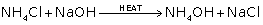d.

i. Haber's process

ii. 1 part of nitrogen gas and 3 parts of hydrogen gas

iii. Finely divided iron (Fe)

iv.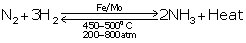e.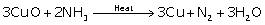f.

i. Ammonia and hydrogen chloride gas

ii. High solubility of gases in waterQuestion 2011

a. State what is observed when - Ammonium hydroxide is first added in a small quantity and then in excess to a solution of copper sulphate.

b. The diagram below shows the setup for the laboratory preparation of a pungent alkaline gas.

i. Name the gas collected in the jar.

ii. Give a balanced equation for the above preparation.

iii. State how the above gas is collected.

iv. Name the drying agent used.

v. State how you will find out that the jar is full of the pungent gas.

c. Write a balanced chemical equation - Chlorine reacts with excess of ammonia.

d. State your observation - Water is added to the product formed when Al is burnt in a jar of nitrogen gas.Solution 2011

a. In small quantity: A bluish white ppt. is obtained.

In excess quantity: A deep blue solution is obtained.

b.

i. Ammonia

ii.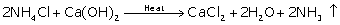iii. By downward displacement of air

iv. Quicklime/CaO

v. Bring a moist red litmus paper to the mouth of  the inverted jar if it immediately turns blue.

Or

Bring a glass rod dipped in hydrochloric acid to the mouth of the inverted jar. If it produces dense white fumes, then the jar is full of gas.

c.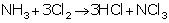d. Pungent smelling basic gas called ammonia is evolved.Question 2012

a. Name - The gas produced when excess ammonia reacts with chlorine.

b. Rewrite the correct statement with the missing word/s: Magnesium nitride reacts with water to liberate ammonia.

c. Give a balanced equation for the reaction: Ammonia and oxygen in the presence of a catalyst.

d. The following questions are based on the preparation of ammonia gas in the laboratory:

i. Explain why ammonium nitrate is not used in the preparation of ammonia.

ii. Name the compound normally used as a drying agent during the process.

iii. How is ammonia gas collected? Explain why it is not collected over water.Solution 2012

a. Nitrogen

b. Magnesium nitride reacts with boiling water to liberate ammonia.

c.d.

i. Ammonium nitrate is a highly explosive substance and cannot be heated.

ii. Quicklime/CaO

iii. By the downward displacement of air or upward delivery as it is lighter than air.

It is not collected over water because it easily dissolves in water.Question 2013

a. State one appropriate observation for: Excess of chlorine gas is reacted with ammonia gas.

b. Nitrogen gas can be obtained by heating

i. Ammonium nitrate

ii. Ammonium nitrite

iii. Magnesium nitride

iv. Ammonium chloride

c. State two relevant observations for: Ammonium hydroxide solution is added to zinc nitrate solution slowly and then in excess.

d. Give a balanced equation for: Reduction of hot copper (II) oxide to copper using ammonia gas.

e. Copy and complete the following table relating to important industrial process:

f. Identify: An alkaline gas which produces dense white fumes when reacted with HCl gas.Solution 2013

a. A yellow-coloured explosive is formed.

b. Ammonium nitrite

c.

i. When added in small quantity, it forms a gelatinous white ppt.

ii. When added in excess, it dissolves to form a complex salt.

d.e.

f.  AmmoniaQuestion 2014

a. Fill in the blank from the choices given in brackets: Ammonia gas is collected by …………. [upward displacement of air, downward displacement of water downward displacement of air].

b. Write a balanced equation for: Action of warm water on magnesium nitride.

c. Distinguish between the following pairs of compounds using the test given in brackets:

i. Iron[II] sulphate and iron[III] sulphate (using ammonium hydroxide)

ii. A lead salt and a zinc salt (using excess ammonium hydroxide)

d. State your observation: Calcium hydroxide is heated with ammonium chloride crystals.

e. Name the other ion formed when ammonia dissolves in water. Give one test that can be used to detect the presence of the ion produced.

f. State the conditions required for: Catalytic oxidation of ammonia to nitric oxide.Solution 2014

a. Downward displacement of air.

b.c.

i. Iron(II) sulphate: Gives dirty green ppt. with ammonium hydroxide insoluble in excess.

ii. Iron(III) sulphate: Gives reddish brown ppt. with ammonium hydroxide insoluble in excess.

d. A colourless pungent-smelling ammonia gas is obtained.

e.

i. Hydroxyl (OH-) ion other than ammonium ion

ii. Red litmus turns blue, methyl orange turns yellow and phenolphthalein turns pink.

f. In the presence of platinum catalyst at 800°C.Question 2015

(a) Give balanced chemical equations for

(i) Lab preparation of ammonia using ammonium salt

(ii) Reaction of ammonia with excess of chlorine

(iii) Reaction of ammonia with sulphuric acid

(b) From the list of gases - ammonia, ethane, hydrogen chloride, hydrogen sulphide, select the gas which is used as a reducing agent in reducing copper oxide to copper.Solution 2015

(a)

(i)  2NH4Cl + Ca (OH)2 → CaCl2 + 2H2O + 2NH3

(ii) NH3 + 3Cl2→ NCl3 + 3HCl

(Nitrogen trichloride)

(iii) 2NH3 + H2SO4→ (NH4)2SO4

Ammonium sulphate

(b) Ammonia (NH3)Question 2016 (a)

Name

(a) Name the gas evolved when the following mixtures are heated :

(i) Calcium hydroxide and Ammonium Chloride

(ii) Sodium Nitrite and Ammonium Chloride Solution 2016 (a)

(i) Ammonia

(ii) Nitrogen Question 2016 (b)

(b) Write balanced chemical equations for each of the following :

(i) When excess of ammonia is treated with chlorine.

(ii) An equation to illustrate the reducing nature of ammonia. Solution 2016 (b)

(i) 8NH3 + 3Cl2→ N2 + 6NH4Cl

(ii) 3PbO + 2NH3→3Pb + 3H2O + N2

error: Content is protected !!